• 2022-03-13 20:36:00

## 1.激活函数

### 1.1 Sigmoid函数

Sigmoid 是常用的非线性的激活函数，表达式如下：
f ( x ) = 1 1 + e − x f(x) = \frac{1}{1 + e^{-x}}

• 特性：它能够把输入的连续实值变换为 0 0 1 1 之间的输出，特别的，如果是非常大的负数，那么输出就是 0 0 ；如果是非常大的正数，输出就是 1 1 .
• 缺点：在深度神经网络中梯度反向传递时导致梯度爆炸和梯度消失，其中梯度爆炸发生的概率非常小，而梯度消失发生的概率比较大。

### 1.2 tanh函数

tanh函数也是非线性函数，其函数解析式为：
t a n h ( x ) = e x − e − x e x + e − x tanh(x) = \frac{e^x - e^{-x}}{e^x + e^{-x}}
tanh读作Hyperbolic Tangent，它解决了Sigmoid函数的不是zero-centered输出问题，然而，梯度消失(gradient vanishing)的问题和幂运算的问题仍然存在。

### 1.3 Relu函数

Relu函数实际上就是个取最大值函数，其函数解析式如下所示：
f ( x ) = max ⁡ ( 0 , x ) f(x) = \max{(0, x)}

• Relu是目前最常用的激活函数，一般搭建人工神经网络时推荐优先尝试
• Relu并非全区间可导，但我们可以取sub-gradient
1. 解决了gradient vanishing问题 (在正区间)
2. 计算速度非常快，只需要判断输入是否大于 0 0
3. 收敛速度远快于Sigmoidtanh
1. ReLU的输出不是zero-centered
2. Dead ReLU Problem，指的是某些神经元可能永远不会被激活，导致相应的参数永远不能被更新。有两个主要原因可能导致这种情况产生: (1) 非常不幸的参数初始化，这种情况比较少见 (2) learning rate太高导致在训练过程中参数更新太大，不幸使网络进入这种状态。解决方法是可以采用Xavier初始化方法，以及避免将learning rate设置太大或使用adagrad等自动调节learning rate的算法。

### 1.4 Leaky ReLU函数(PReLU)

函数表达式：
f ( x ) = max ⁡ ( α x , x ) f(x) = \max(\alpha x, x)
人们为了解决Dead ReLU Problem，提出了将ReLU的前半段设为 α x \alpha x 而非 0 0 ，通常 α = 0.01 \alpha=0.01 。另外一种直观的想法是基于参数的方法，即 P a r a m e t r i c R e L U : f ( x ) = max ⁡ ( α x , x ) Parametric ReLU:f(x) = \max(\alpha x, x) ，其中 α \alpha
可由方向传播算法学出来。理论上来讲，Leaky ReLUReLU的所有优点，外加不会有Dead ReLU问题，但是在实际操作当中，并没有完全证明Leaky ReLU总是好于ReLU

### 1.5 ELU(Exponential Linear Units) 函数

函数表达式：
f ( x ) = { x , i f   x > 0 α ( e x − 1 ) , o t h e r w i s e f(x) = \left\{\begin{matrix} x ,&if\ x > 0\\ \alpha(e^x - 1), &otherwise \end{matrix}\right.
ELU不会有Dead ReLU问题 输出的均值接近 0 0 zero-centered。但计算量偏大，在目前的实际应用中并未被证明总是好于ReLU

### 1.6 UnitStep 阶跃函数

函数表达式：
f ( x ) = { 1 , i f   x > 0 0 , o t h e r w i s e f(x) = \left\{\begin{matrix} 1 ,&if\ x > 0\\ 0, &otherwise \end{matrix}\right.
传统的阶跃函数，不连续，因此难以进行数学分析。常用其它连续可导函数代替。

## 2.感知机模型(神经元模型)

设输入空间(特征空间)为 X ⊆ R n X\subseteq\R^n ，输出空间为 Y = { 0 , 1 } Y = \{0, 1\}

输入 x ∈ X x \in X 为实例的特征向量，输出 y ∈ Y y \in Y 为实例的类别

由输入空间到输出空间的如下函数称为感知机：
f ( x ) = s i g n ( w x + b ) f(x) = sign(wx + b)
其中 w w b b 为模型参数， w ∈ R n w \in \R^n 称为权值， b ∈ R b \in \R 称为偏置。 s i g n sign 是符号函数。

假设我们目前的任务是通过感知机对具有 n n 维特征的向量进行分类。我们可以将该感知机的模型视作一个神经元模型。 n n 维向量( ( x 1 , x 2 , … , x n ) (x_1, x_2, \dots, x_n) )对应的是神经元的 n n 个输入。我们对这 n n 个输入分别乘其对应的权值后求和，经过激活函数后得到分类结果。

但是我们注意到，当神经网络的输入向量为 0 0 时，会产生激活失败误分类的情况，为了避免这种情况，我们对其加偏置项后再进入激活函数，也就是感知机模型中的 b b

我们首先对输入向量乘对应的权值加偏置项后得到 ∑ k = 1 k ≤ n w x i + b \sum_{k = 1}^{k \leq n} wx_i + b ，将其经过激活函数后与标签进行比对，并根据是否与标签相等来更新参数的值：
w = w + α × ( y − y ^ ) × x b = b + α × ( y − y ^ ) w = w + \alpha \times(y - \hat{y})\times x \\ b = b + \alpha \times (y - \hat{y})
其中 α \alpha 为步长，又称学习率。以上过程对所有训练数据执行一次后，可以得到一轮训练后的 w w b b

显然，由于权值参数对应于 n n 维特征向量，因此 w w 的维度一定与输入向量的特征维数有关。

此处给出基于Python实现的感知机模型。

import numpy as np
import matplotlib.pyplot as plt

class ActivateFunction(object):
@staticmethod
def Sigmoid(x):
return (1 / (1 + np.exp(-x)))
@staticmethod
def ReLu(x):
if x <= 0: return 0
else: return x
#return np.max(0, x)
@staticmethod
def Softmax(x):
return np.exp(x) / np.sum(np.exp(x))
@staticmethod
def UnitStep(x):
return 1 if x > 0 else 0

class Perceptron(object):
#初始化一个具有n维特征的感知机
def __init__(self, input_num, activator):
self.activator = activator
self.size = input_num
self.weights = [0.0 for i in range(input_num)]
self.bias = 0.0
#预测值
def Predict(self, input_vec):
return self.activator(np.dot(input_vec, self.weights) + self.bias)
#单轮训练
def SingleIteration(self, input_vecs, labels, rate):
samples = zip(input_vecs, labels)
for (input_vec, label) in samples:
output = self.Predict(input_vec)
delta = label - output
input_vec = np.array(input_vec)
self.weights += rate * delta * input_vec
self.bias += rate * delta
#多轮训练入口
def fit(self, input_vecs, labels, iteration, rate):
input_vecs, labels = np.array(input_vecs), np.array(labels)
for i in range(iteration):
self.SingleIteration(input_vecs, labels, rate)
#获取训练后的得到参数
def GetParameters(self):
return self.weights, self.bias

if __name__ == "__main__":
data, label = [], []
file = open(r'.\Python\x.txt')
line_data = line.strip().split(',')
data.append([float(line_data), float(line_data)])
file.close()
file = open(r'.\Python\y.txt')
line_data = line.strip().split(',')
label = list(map(int, line_data))
file.close

p = Perceptron(2, ActivateFunction.UnitStep)
p.fit(data, label, 1000, 0.1)
w, b = p.GetParameters()
x1 = np.arange(-5, 10, 0.1)
x2 = (w * x1 + b) / (-w)

data = np.array(data)
label = np.array(label)
idx_p = np.where(label == 1)
idx_n = np.where(label != 1)
data_p = data[idx_p]
data_n = data[idx_n]

plt.scatter(data_p[:, 0], data_p[:, 1], color='red')
plt.scatter(data_n[:, 0], data_n[:, 1], color='blue')
plt.plot(x1, x2)
plt.show()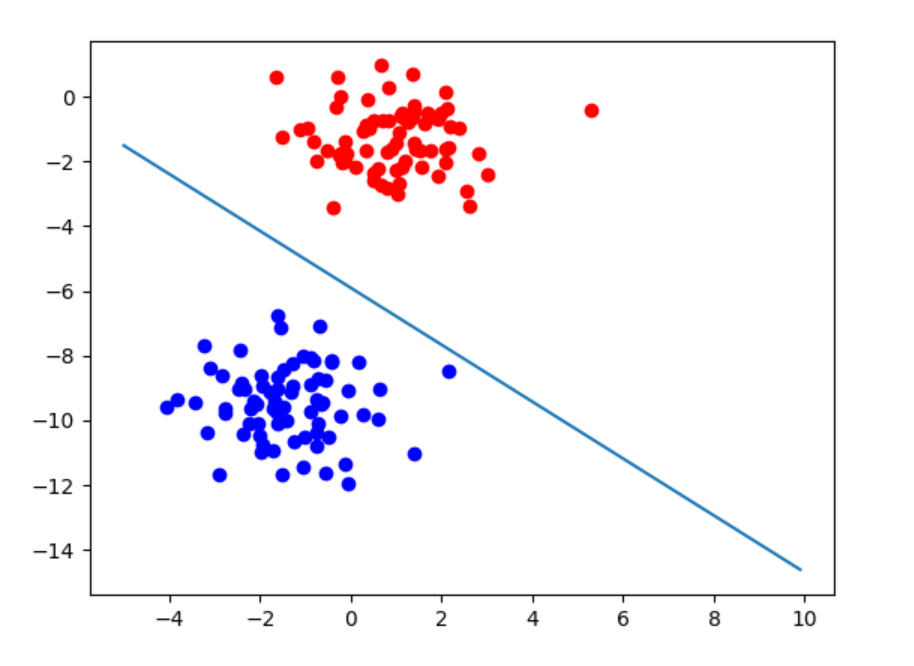分类效果示例如上所示。神经网络 深度学习 机器学习
更多相关内容
• 一、什么是感知机模型？ 感知机是线性分类的二分类模型，输入为实例的特征向量，输出为实例的类别，分别用1和-1表示。感知机将输入空间（特征空间）中的实例划分为正负两类分离的超平面，旨在求出将训练集进行线性...
• BAT算法工程师深入详细地讲解感知机模型的训练，带你轻松入门深度学习!
• import argparse #一个好用的参数传递模型 import numpy as np from sklearn.datasets import load_iris #数据集 from sklearn.model_selection import train_test_split #训练集和测试集分割 from loguru import ...
• 简化的广义多层感知机模型及其学习算法_方宁.pdf
• BAT算法工程师深入详细地讲解感知机模型，带你轻松入门深度学习！
• 人工神经网络中最简单的模型感知机（perceptron）是二分类的线性分类模型，属于监督学习算法,输入为实例的特征向量，输出为实例的类别（取+1和-1）。
• 数据挖掘技术在医疗诊断中的应用——感知机模型诊断心脏病.pdf
• 感知机模型 感知机是一种判别模型，使用于二分类问题，输入为实例的特征向量，输出实例的类别；取-1和+1分被称为负类和正类。。感知机学习主要是求出将训练数据能够进行线性划分的分离超平面，所以就有了基于误分类...

# 感知机模型

感知机模型的对偶形式
感知机是一种判别模型，使用于二分类问题，输入为实例的特征向量，输出实例的类别；取-1和+1分被称为负类和正类。。感知机学习主要是求出将训练数据能够进行线性划分的分离超平面，所以就有了基于误分类的损失函数，利用梯度下降法对损失函 数进行极小化，求得感知机模型 感知机学习算法具有简单而易于实现的优点，分为 原始形式和对偶形式 。

首先我们来看看感知机模型

模型：由输入空间到输出空间的如下函数：
f ( x ) = s i g n ( w • x + b ) f(x) = sign (w•x + b)
sign()为符号函数 ；

w,b:为模型参数（w : 权值或权值向量，b：偏置常量，w•x ：内积（向量相乘再求和为得到 S ，即确定模型参数w,b,定义损失函数并极小化

损失函数：在感知机模型中损失函数的一个自然选择是误分类点的总数。但是，这样的损失函数不是参数w,b的连续可导函数，不易优化。损失函数的另一个选择是误分类点到超平面的总距离，感知机所采用的就是这个损失函数。为此，首先写出输入空间 中任一点 Xo 到超平面距离:||w|| 是 w的L2范数

对于误分类数据（xi,yi）:
− y i ( w • x i + b ) > 0 -yi(w•xi + b) > 0
误分类点xi到S的距离：

• 1 / ||w|| * yi*(w•xi + b)

误分类点特征：
w • x i + b > 0 , y i = − 1 w•xi + b >0, yi = -1
w • x i + b < 0 , y i = + 1 w•xi + b <0,yi = +1

设S的误分类点集合为M，则误分类点到S的总距离：
− 1 / ∣ ∣ w ∣ ∣ ∑ y i ( w • x i + b ) ( x i ∊ M ) -1/||w|| ∑ yi (w•xi + b) (xi ∊ M)
判断误分类点
真 实 值 ∗ 预 测 值 = + 1 正 确 分 类 ； 真实值 * 预测值 = +1 正确分类；

真 实 值 ∗ 预 测 值 = − 1 误 分 类 ； 真实值 * 预测值 = -1 误分类；

不考虑 1 / ||w|| --> 损失函数 :
L ( w , b ) = − ∑ y i ( w • x i + b ) ( x i ∊ M ) L(w,b) = -∑ yi (w•xi + b) (xi ∊ M)

• 选取损失函数最小的模型参数w,b

感知机学习算法：随机梯度下降法，任选一个超平面w0,b0,然后梯度下降法不断地极小化目标函数。极小化过程不是一次使M中所有误分类点的梯度下降，而是一次随机选取一个误分类点使其梯度下降；

损失函数L(w,b)的梯度由：
∇ w L ( w , b ) = − ∑ y i x i ( x i ∊ M ) ∇w L(w,b) = -∑yixi (xi ∊ M)

∇ b L ( w , b ) = − ∑ y i ( y i ∊ M ) ∇b L(w,b) = -∑yi (yi ∊ M)

随机选取误分类点(xi,yi),对w,b进行更新

w ⬅ w+ɧyixi
b ⬅ b+ɧyi

例题：解：构建最优化问题
m i n L ( w , b ) = − ∑ y i ( w • x i + b ) ( x i ∊ M ) min L(w,b) = -∑yi(w•xi + b) (xi ∊ M)代码示例：

import numpy as np
import matplotlib.pyplot as plt

p_x = np.array([[3, 3], [4, 3], [1, 1]])  # 创建数据集     x值
y = np.array([1, 1, -1])  # 标记数据集     1：正类；-1：负类    y值
plt.figure()  # 创建图像

for i in range(len(p_x)):  # 遍历数据集  在图中标记数据集各点
if y[i] == 1:
plt.plot(p_x[i], p_x[i], 'ro')  # 将数据集中正类点标记为红色实心点
else:
plt.plot(p_x[i], p_x[i], 'bo')  # 负类标记为蓝色实心点

w = np.array([1, 0])  # 初始化权重系数w
b = 0  # 初始化偏移常数b
delta = 1  # 步长 1

for i in range(100):
choice = -1  # 初始化标记参数choice
for j in range(len(p_x)):
if y[j] != np.sign(np.dot(w, p_x) + b):  # w与p_x内积 + b > 0 则sign=1;< 0 则sign=-1   （sign == y[i] 正确分类）
choice = j
break
if choice == -1:
break
w = w + delta * y[choice] * p_x[choice]  # 更新参数
b = b + delta * y[choice]

line_x = [0, 10]  # 绘制x坐标系
line_y = [0, 0]

for i in range(len(line_x)):
line_y[i] = (-w * line_x[i] - b) / w      # 绘制超平面

plt.plot(line_x, line_y)
plt.savefig("picture.png")



实现结果：感知机模型的对偶形式

展开全文python 机器学习
• 只要被处理的数据线性可分，就能使用感知机模型训练得到二分类模型。若数据线性不可分，训练会出现模型来回震荡的情况。 单层感知机模型图 感知机的数学表达式：f(x) = sign(wx+b) 其中，x为输入向量，w为输入向量...
1. 感知机（Perceptron）概述

1957年被提出，是一种有单层计算单元的神经网络模型，在结构上与M-P模型相似，提出初衷是解决数据的分类问题。感知机是神经网络和支持向量机的基础。

1. 感知机原理

感知机本身是一种能进行二分类的线性模型。只要被处理的数据线性可分，就能使用感知机模型训练得到二分类模型。若数据线性不可分，训练会出现模型来回震荡的情况。
单层感知机模型图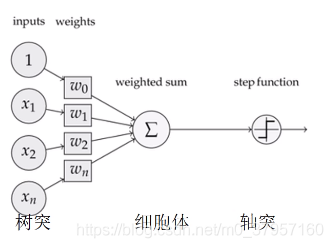感知机的数学表达式：f(x) = sign(wx+b)
其中，x为输入向量，w为输入向量对应权重值，b为偏置，w·x是点积，sign为符号函数，其定义：
s i g n ( x ) = { + 1 ,   x > 0 − 1 ,   x < 0 sign(x) = \begin{cases} +1,\ x>0 \\ -1 ,\ x<0 \end{cases}
由此可得到
s i g n ( ω ⋅ x + b ) = { + 1 ,   ω ⋅ x + b > 0 − 1 ,   ω ⋅ x + b < 0 sign(\omega \cdot x + b) = \begin{cases} +1, \ \omega \cdot x + b>0\\ -1, \ \omega \cdot x + b<0 \end{cases}

如果输入向量x是要进行分类的数据，那么输出结果正负1就可以看作数据经过模型计算后输出的对应标签，这样我们就可以将输入x分为两类。如果处在二维平面中， ω ⋅ x + b = 0 \omega \cdot x + b = 0 对应的直线就是将输入数据二分类的直线，也称为分割超平面（Separating Hyperplane）。

感知机优点：很容易处理线性可分问题，缺点：不能处理异或问题，即不能处理非线性问题。因此出现可处理非线性问题的多层感知机。感知机是一个有监督的学习算法；

1. 感知机学习策略

有了模型之后就需要确定模型的参数；模型的参数就是w和b，即权重和偏置。我们希望找到一个好的w和b，来得到好的分类超平面，能够把所有的样本进行非常好的划分。

做法：实际上学习的策略就是要为感知机模型或者任意一个模型来定义一个损失函数（损失函数就是衡量一下当前模型性能对于已知的样本分类的准不准。有一个训练数据，模型在训练数据上有多少是对的，有多少是错的，那些犯错的地方就是给模型带来损失的地方），怎么衡量一个模型在数据上的损失呢？有两种选择：其一是误分类点的数目。其二是误分类点到超平面的总距离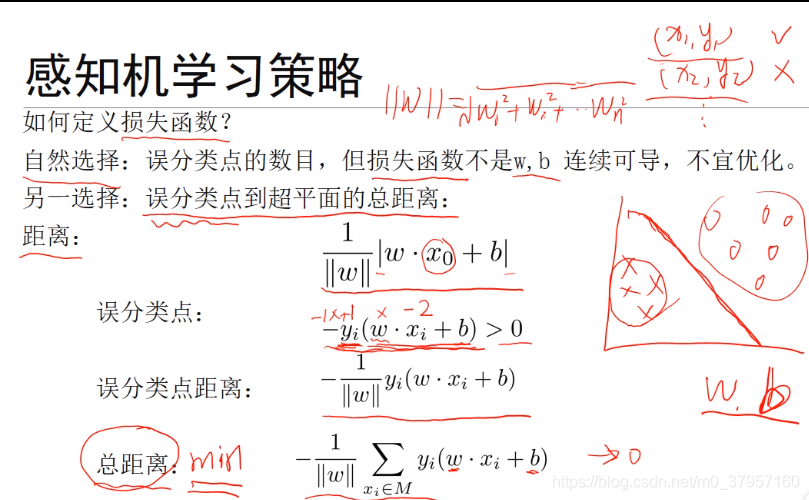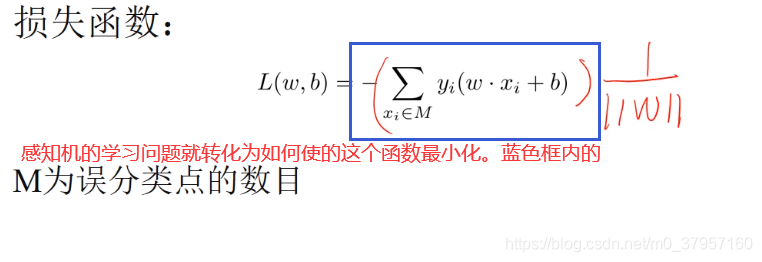感知机的学习问题就转化为如何使的损失函数最小化。
对函数的极值进行求解的时候，最基本的算法叫做梯度下降法。所有的梯度下降法第一步都要初始化。所谓初始化就是把里边未知的参数我要给他赋一个值。对于这里随机的对w和b进行初始化实际上就是随机的选择了一个超平面。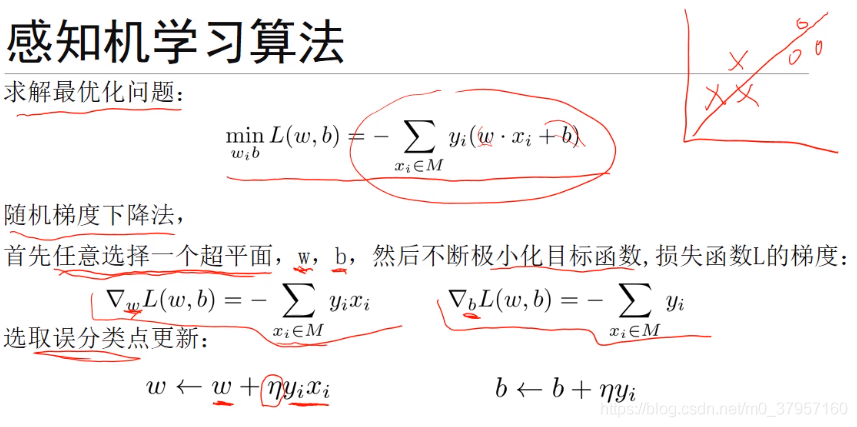其中 η \eta 是我们对参数进行优化放缩的，下降的步长。 η \eta 取的很大，那么他的下降速度就会很快

算法分解：

1、输入；（是训练集和学习率）

对于这个算法来说数据的输入首先要有一个训练的数据集，对于感知机来说他是一个监督学习算法，他是有监督的，所以他的数据都是以输入的向量和他所对应的类别标签形成的一个样本，是第一个样本，一直到是第N个样本，构成了一个包含有N个样本的训练数据集T，其中每一个输入的他都是一个n维的实数向量，是从1到N，有N个样本。他的输出空间是+1或者-1，他本质上是一个二类的分类模型。Binary class。另一个参数学习率也是我们认为指定的，就是我们以一个什么样的学习率来更新我们的参数（这个不是我们学习得来的，而是通过人工经验来设置的一个参数）。

2、输出；（使我们要学习的模型参数w和b）

实际上就是找到f(x)函数，该函数实际上就是确定w和b。有了w和b以后输入x的话我就可以给你算出相应的值来。实际上输出就是这个w和b就是这个权重向量和偏差。
3、算法流程；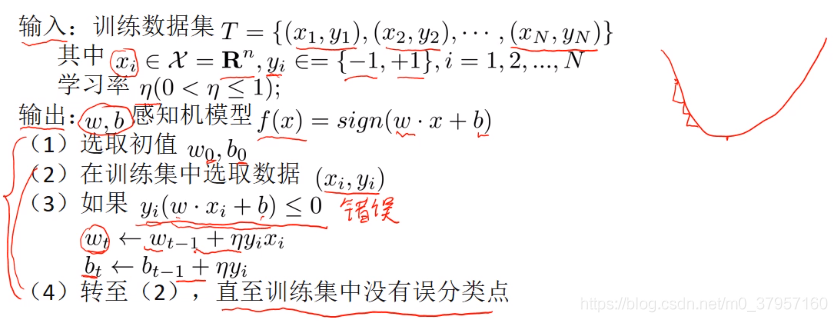（1）初始化w和b。

（2）在训练集当中逐个的选取训练数据的样本，比如说i从1到N逐个的选取。（循环遍历所有的数据样本）

（3）如果选取的样本满足 y i ( ω x + b ) < = 0 y_i( \omega x +b)<=0 ，这就意味着用我当前的w和b，得出来的分类是错了，也就是说我现在这个模型对 ( x i , y i ) (x_i, y_i) 分类是有误的。（整体为负说明计算的这部分结果的符号，和真实的符号是不一致的，所以小于0分类是错误的。（本来两者应该是同号的才表示分类是正确的）)如果分类是错误的，就要更新w和b。更新的方法就是现在的当前的w或者b加上他的偏导乘以学习率，这样就得到一个新的w和b，这就更新了w和b。

（4）更新完w和b之后接下来就是一个循环的过程，就转回到（2），再接着从训练集里边选取新的数据，下一个数据 ( x i , y i ) (x_i,y_i) ，如果他分类错误了我继续还是更新w和b。一直到我把所有的数据都能够正确分类了，直至训练集中没有误分类点，也就是这个算法收敛了。因为模型把所有的训练集都能够正确分类了。那么模型就到此学习过程就结束了。

1. 感知机 Vs 支持向量机

前者最大程度追求正确划分，最小化错误，容易发生过拟合；后者尽量同时避免过拟合
前者的学习策略是最小化损失函数并使用梯度下降法；后者采用的是利用不等式的约束条件构造拉格朗日函数并求极值
前者无最优解，或者说解不唯一

1. 算法实现
原文链接：https://blog.csdn.net/huanyingzhizai/article/details/93525995
import numpy as np
import matplotlib.pyplot as plt

class showPicture:
'''
超平面可视化
'''
def __init__(self, x, y, w, b):
self.b = b
self.w = w
plt.figure()
plt.title('')
plt.xlabel('$x^{(1)}$', size=14)
plt.ylabel('$x^{(2)}$', size=14, rotation = 0)

# 绘制分离超平面
xData = np.linspace(0, 5, 100)
yData = self.expression(xData)
plt.plot(xData, yData, color='r', label='y1 data')

# 绘制数据点
nums = x.shape
for i in range(nums):
plt.scatter(x[i],x[i], c = 'r' if y[i] == 1 else 'b', marker =  '+' if y[i] == 1 else '_', s = 150)

plt.savefig('img/perceptron/incomplement01.png',dpi=75)

def expression(self,x):
'''
根据模型参数预测新的数据点的分类结果
'''
y = (-self.b - self.w*x)/self.w
return y

def show(self):
plt.show()

class perceptron:
'''
感知机模型
'''
def __init__(self,x,y,a=1):
'''
训练数据集X，Y
学习率a设置为1
'''
self.x = x
self.y = y
self.w = np.zeros((x.shape,1))    # 选取初值w0，b0
self.b = 0
self.a = 1

def sign(self,w,b,x):
'''
定义符号函数
'''
y = np.dot(x,w)+b
return int(y)

def train(self, logprint = False):
'''
训练感知机模型
logprint：是否打印每次迭代结果，默认不打印
'''
flag = True
length = len(self.x)
while flag:
count = 0    # 迭代控制
for i in range(length):
tmpY = self.sign(self.w,self.b,self.x[i,:])
if tmpY*self.y[i]<=0:    # 若数据被误分类
tmp = self.y[i]*self.a*self.x[i,:]
tmp = tmp.reshape(self.w.shape)    # 梯度下降ayi_x_i存储为列向量
self.w = tmp +self.w    # 权值更新
self.b = self.b + self.y[i]    # 偏置更新
count +=1
if logprint == True:    # 打印日志
print('第%d次迭代：\n更新后参数 w 为%f，b 为%f' %(count, self.w, self.b))
if count == 0:
flag = False    # 无误分退出迭代
return self.w,self.b

x = np.array([3, 3, 4, 3, 1, 1]).reshape(3, 2)
y = np.array([1, 1, -1])

testp = perceptron(x, y)
w, b = testp.train()

tests = showPicture(x, y, w, b)


展开全文机器学习 深度学习 算法
• 神经网络算法属于机器学习领域中比较热门的内容，而谈到神经网络算法的历史，就不得不提到M-P神经元和基于其构建的感知机模型，虽然该算法有巨大的不足，但是对于我们深入理解复杂的神经网络也是很有帮助的，为此...

# 一.前言

神经网络算法属于机器学习领域中比较热门的内容，而谈到神经网络算法的历史，就不得不提到M-P神经元和基于其构建的感知机模型，虽然该算法有巨大的不足，但是对于我们深入理解复杂的神经网络也是很有帮助的，为此本文聚焦于感知机模型的解读及其实现，话不多说，请看下文。

# 2.1 神经元

在正式介绍感知机前，需要先了解神经元的概念。我们知道，生物神经网络是由一个个神经元构成的，每个神经元都具有多个树突和一个轴突，其中树突用来接受信息，轴突可以通过尾端的轴突末梢（即突触）跟其他神经元的树突连接进行信号的传递。

神经元之间的信息传递属于化学物质传递。当神经元兴奋时，其会向与其相连的神经元发送化学物质，从而改变这些神经元内的电位，当其电位超过一定的阈值后，神经元便会被激活，即兴奋起来，然后向其它神经元发送化学物质。图1：神经元结构

注：图1来源于神经元的维基百科。

1943年，McCulloch和Pitts参考生物的神经元结构，提出了M-P神经元模型。图2中展示的便是M-P神经元，从图中便可以看出，该模型可以接收其他神经元输入的信号，这些信号通过带权重的连接进传递，神经元接受到的总输入信号会与神经元的阈值进行比较，然后通过激活函数处理以产生神经元的输出。图2：M-P神经元模型

注：图2来源于周志华《机器学习》的图5-1。

对于M-P神经元，其使用的激活函数为阶跃函数，其将输入映射为输出值0（神经元抑制）或1（神经元兴奋），其数学定义为：
sgn ( x ) = { 1 , x ≥ 0 0 , x < 0 (1) \text{sgn}(x) = \begin{cases} 1,x \geq 0 \\ 0, x < 0 \end{cases} \tag{1}

阶跃函数的数学性质不太好，例如不连续，因此经常使用Sigmoid来进行替代。

# 2.2 感知机

感知机（Perceptron）模型包含两层神经元，即输入层输出层（示例参见图3），其中输入层接受外界信号后传递给输出层，输出层为M-P神经元。图3：感知机模型示例

感知机模型的数学形式为：
y = f ( ∑ i w i x i − θ ) (2) y = f(\sum_{i}w_ix_i - \theta) \tag{2}
其中 f f 表示阶跃函数 x i x_i w i w_i 分别表示第 i i 个输入及其对应的输入权重 θ \theta 表示阈值。感知机模型中的权重参数 w i w_i 和阈值参数 θ \theta 都可以通过学习得到，其中阈值 θ \theta 可以看作一个固定输入为 − 1.0 -1.0 的哑结点所对应的连接权重。

感知机的学习规则为：对训练样例 ( x , y ) (\bold{x},y) ，若当前感知机的输出为 y ^ \hat{y} ，则感知机将这样调整权重：
w i ← w i + Δ w i Δ w i = η ( y − y ^ ) x i (3) w_i \leftarrow w_i + \varDelta w_i \\ \varDelta w_i = \eta (y - \hat{y})x_i \tag{3}
其中 η ∈ ( 0 , 1 ) \eta \in (0,1) 被称之为学习率，从上述公式可知，当模型的预测正确时， Δ w i = 0 \varDelta w_i=0 ，此时感知机将不发生变化，否则将根据错误的程度进行权重调整。

感知机只能处理线性可分的问题，这是其致命的缺陷，当年也正是关于这个缺陷的证明，使得深度学习进入第一个”寒冬“。

# 三.具体实现

## 3.1 数据集构造

为了可视化方便，本算法拟构造二维数据集，数据集中的样本分为两类，一类的标签为 1 1 ，另一类的标签为 0 0 ，下面是构造数据集的源码：

def generateDataset(num_samples, loc = 4, scale = 2, num_features = 2):
"""
生成二分类数据集
num_samples: 样本数
num_features: 样本的特征数
"""
size = num_samples // 2
# 生成样本
x1 = np.random.normal(loc, scale, (size, num_features))
x2 = np.random.normal(-loc, scale, (num_samples - size, num_features))
x = np.vstack((x1, x2))
# 生成标签
y = np.zeros(num_samples)
y[:size] = 1
# 打乱数据集
indices = np.arange(num_samples)
np.random.shuffle(indices)
x = x[indices]
y = y[indices]

return x,y


构造好数据集后，还对数据集进行了打乱操作。

## 3.2 模型实现、训练及结果

根据第二节中的描述，我们很容易就可以实现一个感知机模型：

class Perceptron():
def __init__(self,in_feats) -> None:
# + 1 means \theta
self.w = np.random.randn(1, in_feats + 1) * 0.01

def sgn(self,x):
"""
阶跃函数
"""
return int(x >= 0)

def forward(self,x):
"""
x: 样本 (num_features, 1)
"""
y_hat = np.dot(self.w,x)
return self.sgn(y_hat)

def update(self,y_hat,y,lr,x):
"""
权重调整
y_hat: 预测值
y: 真实标签
lr: 学习率
x: 样本 (num_features, 1)
"""
self.w += lr * (y - y_hat) * x.T


在实现的感知机模型中需要传入样本的特征数，在实验过程中，先调用数据集构造函数先生成一个包含1000个样本的二维点集，某次实验中生成的数据集可视化如下图4所示。图4：原始数据集

然后需要创建Perceptron类的实例并进行模型的训练，实现的完整源码如下所示：

from data import generateDataset
import matplotlib.pyplot as plt
from model import Perceptron
import numpy as np

def train(model,x,y,lr,accuracy=None):
flag = True
epoch = 0
while flag:
epoch += 1
count = 0
for i in range(x.shape):
y_hat = model.forward(x[i, :].reshape(-1, 1))
if y[i] != y_hat:
model.update(y_hat, y[i], lr, x[i, :].reshape(-1, 1))
count += 1
acc = 1 - count / x.shape
print("Epoch {}: Accuracy: {:.4f}".format(epoch, acc))
# 完全分类正确或分类的准确率达到设定的标准
if not count or ( accuracy and acc >= accuracy):
flag = False

if __name__ == "__main__":
loc = 5
scale = 2
num_samples = 1000
num_features = 2
lr = 0.01
x,y = generateDataset(num_samples, loc, scale, num_features)
# 生成数据的可视化
plt.figure()
plt.scatter(x[:,0],x[:,1],c=y)
plt.savefig("org_dataset.png")
plt.show()
# 添加阈值对应的固定输入列
neg_ones = -np.ones((num_samples, 1))
x = np.hstack((x,neg_ones))
# 初始化模型
model = Perceptron(in_feats=num_features)
# 训练
train(model, x, y, lr)
w1,w2,theta = model.w.flatten()
x1 = np.linspace(-10, 10, 1000)
y1 = (-w1 * x1 + theta) / w2
plt.figure()
plt.scatter(x[:,0],x[:,1],c=y)
plt.plot(x1,y1,c='r')
plt.savefig("outcome.png")
plt.show()


训练后的结果展示如下：图5：划分超平面与原始数据集展示

结论：可见，经过训练感知机模型能够成功学得一个将线性可分的数据集划分的超平面。但在实际中，完全线性可分是很难做到的，因此可以在训练过程中设置一个结果指标，当训练的结果达到该指标即可，而不必过于苛求完美（源码也由对应的实现）。

# 四.结语

参考文献：《机器学习》——周志华

以上便是本文的全部内容，要是觉得不错的话，可以点个赞或关注一下博主，后续还会持续带来各种干货，当然要是有问题的话也请批评指正！！！

展开全文机器学习 神经网络 深度学习
• 一、多层感知机简介 Softmax回归可以算是多分类问题logistic回归，它和神经网络的最大区别是没有隐含层。理论上只要隐含节点足够多，即时只有一个隐含层的神经网络也可以拟合任意函数，同时隐含层越多，越容易拟合...
• 文章目录感知机（Perception）1 感知机模型2 感知机模型的几何解释3 感知机模型的损失函数4 感知机模型的对偶形式 感知机（Perception） 感知机1957年由Rosenblatt提出，是神经网络与支持向量机的基础。 感知机...机器学习
• 机器学习之感知机模型写在前面感知机模型的初步理解自我理解 写在前面 这部分主要是基于李航老师的《统计学习方法》以及参考部分博客完成，写出来让自己更好理解。 感知机模型的初步理解 感知机模型应该是机器学习中...算法 python 机器学习 人工智能
• 感知机，也叫单层神经网络，是最基础的神经网络模型结构。学习 神经网络 深度学习
• ## 感知机模型详解

千次阅读 2020-04-30 16:39:39
感知机是二分类的线性分类器，其输入为实例的...感知机模型 模型 输入空间：X⊆Rn\mathcal X\sube \bf R^nX⊆Rn 输出空间：Y={+1,−1}\mathcal Y=\{+1,-1\}Y={+1,−1} 决策函数：f(x)=sign(w⋅x+b)f(x)=sign (w\cdot...算法 机器学习 深度学习
• 感知机模型的对偶形式 点击链接可了解简单感知机模型推导过程及其实现python代码 [https://blog.csdn.net/weixin_52762273/article/details/124027702] 感知机模型的对偶形式也称累积形式，相对于之前的感知机多了一...python 机器学习
• 导致这次停更时间有点长了，但是经过了这半年的学习，也学了不少东西，思考后决定，先把李航的《统计学习》这本书上的算法一一代码实现一下，来检测一下是否真的学会了，所以先分享给大家的是第二章：感知机模型！...机器学习 算法 r语言
• BAT算法工程师深入详细地讲解感知机模型解决逻辑运算问题，带你轻松入门深度学习!
• 文章目录感知机（perceptron）线性可分数据集原始形式统计学习方法三要素算法流程对偶形式 感知机（perceptron） 线性可分数据集 原始形式 统计学习方法三要素 模型：y=sign(ωTx+b)y=sign(\omega ^{T}x+b)...机器学习 统计学习方法 perceptron
• 感知机模型的一般形式： 其中，x表示特征向量，和b是感知机模型参数，叫做权值或权值向量，b叫做偏置（bias），表示和x的内积，sign是符号函数，即 感知机模型对应于特征空间中的分离超平面： 感知机对应于特征...机器学习
• 下图为一个简单的单层感知机模型 左侧为输入层，对于所有输入xxx，上标0表示第0层（即输入层），下标0~N表示有N+1个元素。对于中间的权重wijw_{ij}wij​，iii表示上一层的节点编号，jjj表示下一层的节点编号。后面...深度学习 pytorch 神经网络
• 具有多层输出的感知机如下图所示： 图中节点上的符号表示的含义是： xk1x^1_kxk1​代表第1层上的第k个节点 Ok1O^1_kOk1​同样代表第1层上的第k个节点 若能求出∇E∇wjk\frac{\nabla E}{\nabla w_{jk}}∇wjk​∇E​...深度学习 神经网络 pytorch...